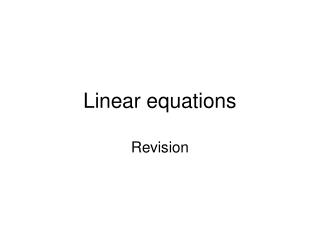DownloadDownload PresentationLinear equations

# Linear equations

Télécharger la présentation## Linear equations

- - - - - - - - - - - - - - - - - - - - - - - - - - - E N D - - - - - - - - - - - - - - - - - - - - - - - - - - -
##### Presentation Transcript

1. Linear equations Revision

2. 4 kinds Easy

3. 4 kinds Easy Two step

4. 4 kinds Easy Two step X on both sides

5. 4 kinds Easy Two step X on both sides Brackets

6. Easy examples

7. Easy examples

8. Easy examples What is the 3 doing?

9. Easy examples What is the 3 doing? Multiplying the x

10. Easy examples What is the 3 doing? Multiplying the x

11. Easy examples What is the 3 doing? Multiplying the x Do the opposite!

12. Easy examples What is the 3 doing? Multiplying the x Do the opposite! Divide by 3

13. Easy examples What is the 3 doing? Multiplying the x Do the opposite! Divide by 3

14. Easy examples

15. Easy examples

16. Easy examples What is the 6 doing?

17. Easy examples What is the 6 doing? Adding to the x

18. Easy examples What is the 6 doing? Adding to the x Do the opposite!

19. Easy examples What is the 6 doing? Adding to the x Do the opposite! Subtract 6

20. Easy examples What is the 6 doing? Adding to the x Do the opposite! Subtract 6

21. Easy examples

22. Easy examples

23. Easy examples What is the 4 doing?

24. Easy examples What is the 4 doing? Dividing the x

25. Easy examples What is the 4 doing? Dividing the x Do the opposite!

26. Easy examples What is the 4 doing? Dividing the x Do the opposite! Multiply by 4

27. Easy examples What is the 4 doing? Dividing the x Do the opposite! Multiply by 4

29. Your turn X=32 X=9 X=4

30. Two step equations

31. Two step equations

32. Two step equations Undo the +4

33. Two step equations Undo the +4

34. Two step equations Undo the +4 Undo the x3

35. Two step equations Undo the +4 Undo the x3

36. Two step equations

37. Two step equations

38. Two step equations Undo the -3

39. Two step equations Undo the -3

40. Two step equations Undo the -3 Undo the x2

41. Two step equations Undo the -3 Undo the x2

43. Your turn X = 5 X = 6 X = 4

44. X on both sides

45. X on both sides

46. X on both sides Take 3x off both sides

47. X on both sides Take 3x off both sides

48. X on both sides Take 3x off both sides Undo -4

49. X on both sides Take 3x off both sides Undo -4

50. X on both sides Take 3x off both sides Undo -4 Undo x2